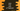# Java program to find the area of a Trapezoid## Java program to find the area of a Trapezoid:

In this post, we will learn how to find the area of a Trapezoid based on user input base and height values.

We will learn different ways to find the area of a Trapezoid.

### Area of a Trapezoid:

• If a and b are bases of a Trapezoid and h is the altitude, it’s area is (a + b)/2 * h.
• So, if we get to know the values of both bases and altitude, we can find out its area.
• Our program will read the values of both bases and altitude as input from the user and print out the area.

### Method 1: Java program to find the area of a Trapezoid:

In this program, we will take the bases and altitude as inputs from the user and print out the are of the Trapezoid.

``````import java.util.Scanner;

public class Main {

public static void main(String[] args) {
double a, b, h;
Scanner sc = new Scanner(System.in);

System.out.println("Enter the first base value : ");
a = sc.nextDouble();

System.out.println("Enter the second base value : ");
b = sc.nextDouble();

System.out.println("Enter the altitude value : ");
h = sc.nextDouble();

double area = (a + b) / 2 * h;

System.out.println("Area : " + area);
}
}``````

Here,

• a, b and h are double values to store the first base, second base and altitude.
• It asks the user to enter the first, second base and altitude values and stores it in a, b and h.
• It calculates the area using the same formula we have seen above and keep that in the variable area.
• At the end, it prints the value of area to the user.

It will give output as like below:

``````Enter the first base value :
10
Enter the second base value :
12
Enter the altitude value :
13
Area : 143.0``````

### Method 2: Java program to find the area of a Trapezoid using a different method:

We can also use a different method to find the area and call it from the main method.

Below is the complete program:

``````import java.util.Scanner;

public class Main {
public static double findArea(double a, double b, double h) {
return (a + b) / 2 * h;
}

public static void main(String[] args) {
double a, b, h;
Scanner sc = new Scanner(System.in);

System.out.println("Enter the first base value : ");
a = sc.nextDouble();

System.out.println("Enter the second base value : ");
b = sc.nextDouble();

System.out.println("Enter the altitude value : ");
h = sc.nextDouble();

System.out.println("Area : " + findArea(a, b, h));
}
}``````

It will give a similar output.

### Method 3: Java program to find the area of a Trapezoid using a different class:

We can also use a different class to keep the height, width and are of the Trapezoid.

Create a file Trapezoid.java with the below code:

``````public class Trapezoid {
double a, b, h;

double findArea(){
return (a + b) / 2 * h;
}
}``````

We can use this class from the main class:

``````import java.util.Scanner;

public class Main {

public static void main(String[] args) {
Trapezoid t = new Trapezoid();
Scanner sc = new Scanner(System.in);

System.out.println("Enter the first base value : ");
t.a = sc.nextDouble();

System.out.println("Enter the second base value : ");
t.b = sc.nextDouble();

System.out.println("Enter the altitude value : ");
t.h = sc.nextDouble();

System.out.println("Area : " + t.findArea());
}
}``````

As you can see here, it creates one Trapezoid object and uses this object to store the base and altitude values. Also, we are calculating the area inside this class.

It gives output similar to the above programs.